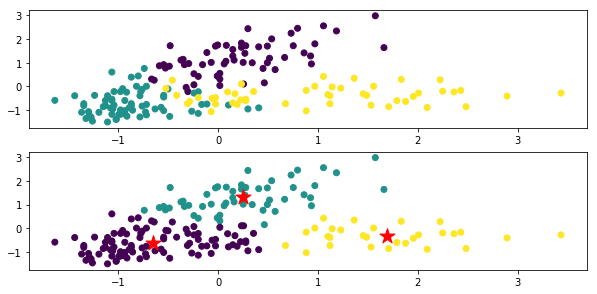# k-means system summary

--k-means: Minimize the squared error from the center of gravity of the cluster. --k-medoids: Perform the EM procedure so that the sum of dissimilarities from the cluster medoids (points belonging to the cluster that minimize the sum of dissimilarities) is minimized. --x-means: Controls cluster division based on BIC. --g-menas: Control cluster division by Anderson darling test, assuming the data is based on a normal distribution. --gx-means: The above two extensions. --etc (See the readme of pyclustering. There are various)

# Determining the number of clusters

It would be nice if humans could see the data immediately and know the number of clusters, but that is rare, so I want a quantitative judgment method.

According to sklearn cheat sheet

Is recommended.

Is also useful, but in my experience, it was rare for me to get a beautiful elbow (a point where the graph becomes jerky), and I was often confused about the number of clusters.

There is x-means as a method of clustering with the number of clusters fully automatically.

Below, how to use the library "pyclustering" that contains various clustering methods including x-means.

# How to use pyclustering

pyclustering is a library of clustering algorithms implemented in both python and C ++.

## Installation

Dependent packages: scipy, matplotlib, numpy, PIL

``````pip install pyclustering
``````

## x-means usage example

Source code

In addition to the EM step in k-means, x-means determines a new step: whether it is appropriate for a cluster to be represented by two or one normal distributions, and two are If appropriate, the operation is to divide the cluster into two.

Below, jupyter notebook is used.

``````import numpy as np
import pandas as pd
import matplotlib.pyplot as plt
from sklearn import cluster, preprocessing

#Wine dataset
#Variety(Row 0, 1-3)And color (10 rows) and amount of proline(13 rows)To use
df_wine=df_wine_all[[0,10,13]]
df_wine.columns = [u'class', u'color', u'proline']

#Data shaping
X=df_wine[["color","proline"]]
sc=preprocessing.StandardScaler()
sc.fit(X)
X_norm=sc.transform(X)

#plot
%matplotlib inline

x=X_norm[:,0]
y=X_norm[:,1]
z=df_wine["class"]
plt.figure(figsize=(10,10))
plt.subplot(4, 1, 1)
plt.scatter(x,y, c=z)
plt.show

# x-means
from pyclustering.cluster.xmeans import xmeans
from pyclustering.cluster.center_initializer import kmeans_plusplus_initializer
xm_c = kmeans_plusplus_initializer(X_norm, 2).initialize()
xm_i = xmeans(data=X_norm, initial_centers=xm_c, kmax=20, ccore=True)
xm_i.process()

#Plot the results
z_xm = np.ones(X_norm.shape)
for k in range(len(xm_i._xmeans__clusters)):
z_xm[xm_i._xmeans__clusters[k]] = k+1

plt.subplot(4, 1, 2)
plt.scatter(x,y, c=z_xm)
centers = np.array(xm_i._xmeans__centers)
plt.scatter(centers[:,0],centers[:,1],s=250, marker='*',c='red')
plt.show
``````The top is a figure colored for each original data class, and the bottom is the clustering result by x-means. The ★ mark is the center of gravity of each class.

In the code `xm_c = kmeans_plusplus_initializer (X_norm, 2) .initialize ()`, the initial value of the number of clusters is set to 2, but it clusters properly to 3.

I am running x-means with `xm_i.process ()`.

For the x-means instance (`xm_i` in the above code), if you look at the instance variables before and after learning, you can see what the learning result looks like. For example

``````xm_i.__dict__.keys()
``````

Or

``````vars(xm_i).keys()
``````

Can be obtained with

``````dict_keys(['_xmeans__pointer_data', '_xmeans__clusters', '_xmeans__centers', '_xmeans__kmax', '_xmeans__tolerance', '_xmeans__criterion', '_xmeans__ccore'])
``````

I think you should look at various things such as.

_xmeans__pointer_data

A copy of the data to be clustered.

_xmeans__clusters

A list showing which line of the original data (\ _xmeans__pointer_data) belongs to each cluster.

The number of elements in the list is the same as the number of clusters, each element is also a list, and the number of the line belonging to the cluster is stored.

_xmeans__centers

A list consisting of the coordinates (list) of the centroid of each cluster

_xmeans__kmax

Maximum number of clusters (set value)

_xmeans__tolerance

A constant that defines the stop condition for x-means iteration. The algorithm terminates when the maximum change in the center of gravity of the cluster falls below this constant.

_xmeans__criterion

It is a judgment condition of cluster division. Default: BIC

_xmeans__ccore

This is the setting value for whether to use C ++ code instead of python code.